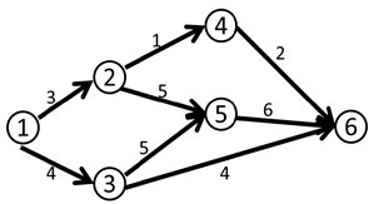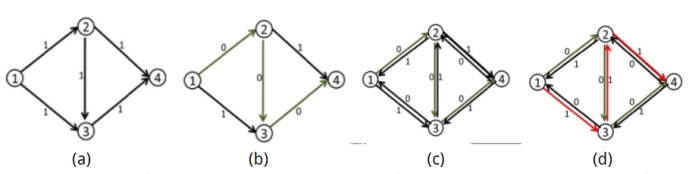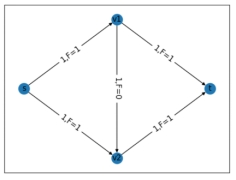# 网络流（Network Flow）基础及Python实现

wuchangjian2021-11-16 17:38:46编程学习

Python networkx 实现网络流_Ugly Gardon-CSDN博客

networkx求解最小费用最大流并可视化数据_engineoid的博客-CSDN博客

• 结点：在该点，流入的流量与流出的相同
• 源点（source）：在该点，流出的流量大于流入的流量
• 汇点（sink）：在该点，流入大于流出## 网络的最大流问题 and The Edmonds-Karp algorithm## 现在如何解决这个问题？

1955年,Harris在研究铁路最大通量时首先提出在一个给定的网络上寻求两点间最大运输量的问题。1956年, Ford等人给出了解决这类问题的标号法，从而建立了网络流理论。现在我们如上个章节一般复杂地求解网络流问题，通过Python的第三方库NetworkX就可以快速地建立网络并求解。``````import networkx as nx
import matplotlib.pyplot as plt

# 创建一个空的有向图
G = nx.DiGraph()

#绘制图
('s','v2',{'capacity': 1}),
('v1','v2',{'capacity': 1}),
('v1','t',{'capacity': 1}),
('v2','t',{'capacity': 1})])

pos=nx.spring_layout(G)#力导向布局算法默认分配的位置
#初始化,选定节点的初始位置/固定节点
pos['t']=1;pos['t']=0
pos['s']=-1;pos['s']=0
pos['v1']=0;pos['v1']=1
pos['v2']=0;pos['v2']=-1

#显示graph
#处理边上显示的容量
edge_label = nx.get_edge_attributes(G,'capacity')

#将节点、边的容量标签，边 这三个元素加入图中
nx.draw_networkx_nodes(G,pos)
nx.draw_networkx_labels(G,pos)
nx.draw_networkx_edges(G,pos)
nx.draw_networkx_edge_labels(G, pos,edge_label,font_size=15)#显示原图像

#求解最大流
maxFlow = nx.maximum_flow(G, 's', 't')
# print(maxFlow)#可以输出流信息看看
maxFlow_const=maxFlow

#取出每条边流量信息存入边显示值
for i in maxFlow_const.keys():
for j in maxFlow_const[i].keys():
edge_label[(i,j)] = str(edge_label[(i,j)]) + ',F=' + str(maxFlow_const[i][j])

#将新的边的标签加入图中
nx.draw_networkx_edge_labels(G, pos,edge_label,font_size=12)

#显示流量及原图
plt.axis('on')
plt.xticks([])
plt.yticks([])
plt.show()
``````

### 青海大通山洪受灾382户居民恢复正常用电

2022-08-20 11:02:49 记者从国网青海省电力公司了解到...

...

...

### 最适合练手的第一个Qt小程序，所有代码均可复制

...

#### 发表评论◎欢迎参与讨论，请在这里发表您的看法、交流您的观点。